# ActinCycle1

Model number
0361

ODE model of actin polymerization and depolymerization with tracking of bound nucleotide

## Description

```This ordinary differential equation model describes actin
polymerization and depolymerization with rates which depend on the
hydrolysis state of bound nucleotide. The model accounts for the
within filaments, the fraction of subunits at each location throughout
the filament which are bound to ATP, ADP-Pi, or ADP. The identity of
bound nucleotide is important for actin because it affects
polymerization and depolymerization kinetics as well as the binding
of several important binding proteins, including ADF/cofilin and
Arp2/3 complex. The published model the current model was based on
was the first to quantitatively identify the distribution of bound
nucleotide along the length of actin filaments. All rate constants
are adjustable, as are capping fractions for each end of the filament.
The model output values include concentrations for all the nucleotide
binding states for monomers, filament concentrations, and nucleotide
binding state fractions for each individual subunit position throughout
the simulated filaments. In addition, the fractions are assembled into
profile variables in order to make it easier to view complete filament
nucleotide profiles.  These profile variables (Pt,Pdpi,Pd) are functions
of two dimensions, s, which is subunit location within filaments measured
from the barbed end, and t, time. So, a plot page curve of Pt(s,100) will
show how the fraction of subunits bound to ATP varies along the entire
filament after 100 seconds of simulation. A plot page curve of Pt(10,t),
on the other hand, will show how the fraction of subunits bound to ATP at
the 10th subunit from the barbed end varies over time.
In the JSim realization of this model, we need a fixed number of
equations and therefore need to fix the length of the filaments we are
simulating. If you would like to investigate filaments of a different length
MPC utility (available at imagwiki.nibib.nih.gov/physiome/jsim/models/webmodel/NSR/MPC/).
If you are interested in this option, make sure that you download the
ActinCycle1.mpc file for this model as well as the ActinCycle1.proj file,
since you will need the MPC source code to generate a new model file with
the correct number of equations for your desired filament length.
Detailed instructions can be found in the model source code and in
the MPC source code. Note that doubling the length of the simulated
filaments increases the simulation computation time by a factor
of 5 to 6.```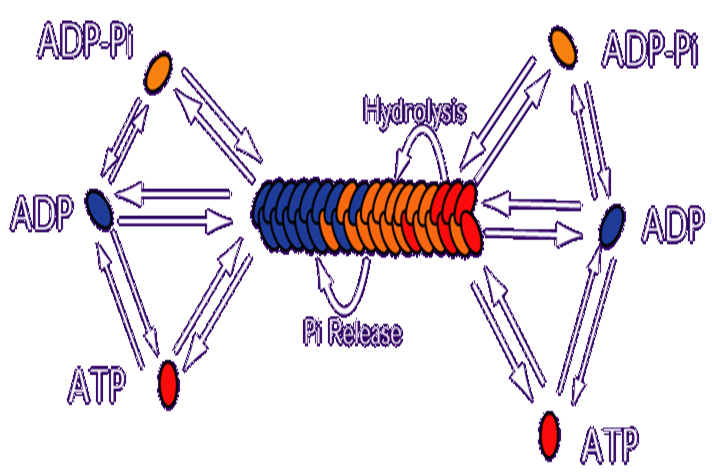## Equations

#### ODEs governing changes in monomer concentrations: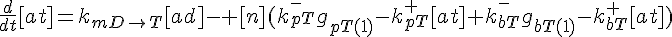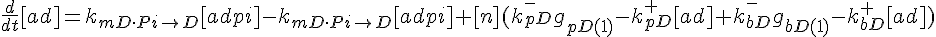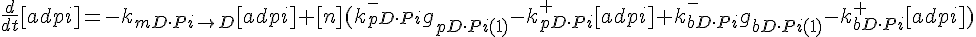#### Flow into and out of filamentous pool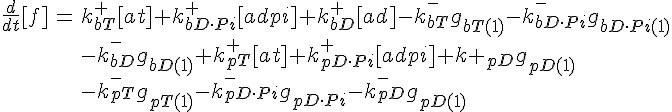#### Conservation of Actin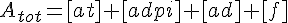#### ODEs governing filament tip subunit fractions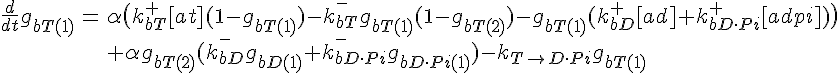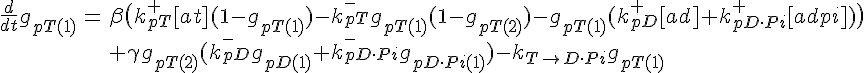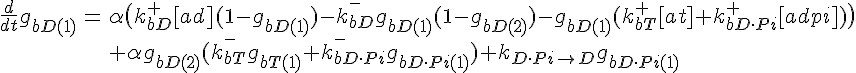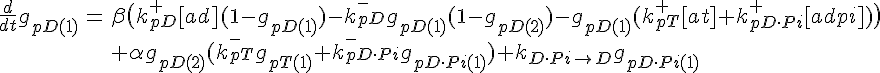Filament tip subunit fractions must sum to one: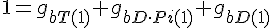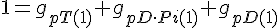#### Equations governing internal subunit fractions

The following are the equations for the ith subunit from the tip, counting from the barbed end: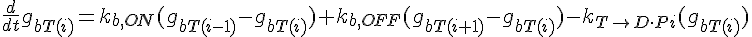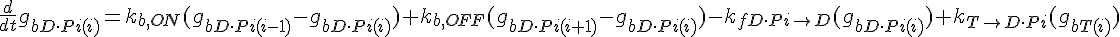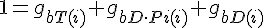where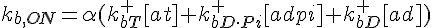,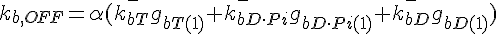The following are the equations for the ith subunit from the tip, counting from the pointed end: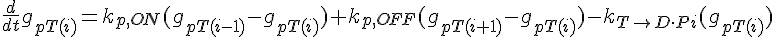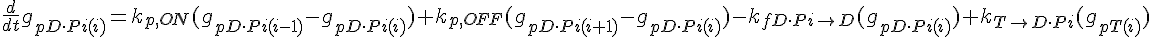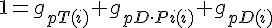where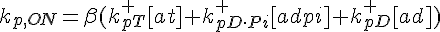,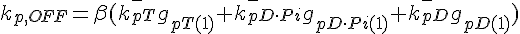For the subunit at the exact center of the filament (designated here by location i*), we require that it lies on a cubic spline connecting subunits from the barbed and pointed end governed equations. This guarantees that the value of the profile and it's derivative vary smoothly across this center connection point.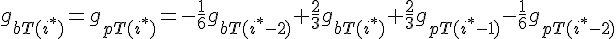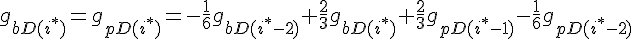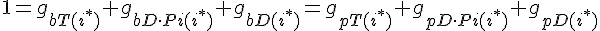The equations for this model may be viewed by running the JSim model applet and clicking on the Source tab at the bottom left of JSim's Run Time graphical user interface. The equations are written in JSim's Mathematical Modeling Language (MML). See the Introduction to MML and the MML Reference Manual. Additional documentation for MML can be found by using the search option at the Physiome home page.